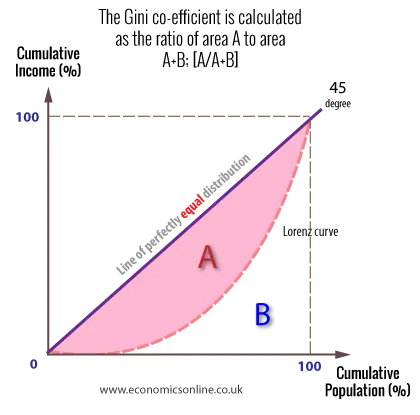# The Gini co-efficient

#### Gini co-efficient

The gini co-efficient is a measure of inequality in an economy – the higher the value, the greater the inequality.

It can be derived by comparing areas on a Lorenz curve, as follows:If there is a perfectly equal distribution of income the whole area will be B, hence A/A + B will give us zero. When there is perfect inequality, the whole area will be A, hence A/A+B give the value of one. So the higher the number between zero and one, the greater the inequality.